Notebook

This notebook features the Quantopian-based research presented in the paper "Momentum with Volatility Timing". Specifically, the paper proposes two extensions to the conventional momentum factor strategy by introducing the volatility-timed winners approach. First, for resolving factor underperformance in the 2011-2018 post-crisis period, the conventional winners-minus-losers momentum is replaced with the winners-only component. Second, the proposed approach substitutes constant volatility scaling with the threshold function and uses past volatilities as a timing predictor for changing momentum strategies. The approach was confirmed with Spearman rank correlation and demonstrated in relation to different strategies including momentum volatility scaling, risk-based asset allocation, time series momentum and MSCI momentum indexes.

The notebook is structured as follows:

Part I: Analysis and Approaches Presented in the Paper

Part II: Paper-Related Techniques and Extensions Proposed within this Forum
In :
import datetime
import numpy as np
import pandas as pd
from pandas.tseries.offsets import BDay
import matplotlib.pyplot as plt
from quantopian.pipeline import Pipeline
from quantopian.pipeline.data.builtin import USEquityPricing
from quantopian.pipeline.factors import CustomFactor, Returns, DailyReturns
from quantopian.research import run_pipeline
import alphalens as al
import math

In :
import pyfolio as pf
import empyrical as ep


# Part I: Analysis and Approaches Presented in the Paper¶

## 1. Quantopian and Alphalens Processing¶

This section is based on the Quantopian and Alphalens framework that is comprehensively described in Lecture 39: Factor Analysis with Alphalens. In comparisson with the lecture, however, the paper applies the Quantopian framework to the conventional winners-minus-losers momentum strategy that was documented by Jegadeesh and Titman (1993). The corresponding factor is computed by ranking stocks according to their prior behavior over the course of 11 months with a 1 month lag:

In :
class Momentum(CustomFactor):
""" Conventional Momentum factor """
inputs = [USEquityPricing.close]
window_length = 252

def compute(self, today, assets, out, prices):
out[:] = (prices[-21] - prices[-252])/prices[-252]


Defining time interval covering the 2008-2009 market downturn

In :
start_date, end_date = '2004-07-01', '2010-12-31'


Running pipeline with Momentum class for calculating momentum prior scores

In :
universe = QTradableStocksUS()

def make_pipeline():

pipe = Pipeline()
pipe.set_screen(universe)

return pipe

pipe = make_pipeline()
results = run_pipeline(pipe, start_date, end_date)
results.dropna(inplace=True)

mom_scores = results['momentum']


Pipeline Execution Time: 22.15 Seconds

Retrieving prices for pipeline assets

In :
asset_list = results.index.levels
prices = get_pricing(asset_list, start_date=start_date, end_date=end_date, fields='open_price')


Calculating forward returns and quantile ranks with Alphalens

In :
mom_data = al.utils.get_clean_factor_and_forward_returns(factor=mom_scores,
prices=prices,
quantiles=10,
periods=(1, 21))

Dropped 1.5% entries from factor data: 1.5% in forward returns computation and 0.0% in binning phase (set max_loss=0 to see potentially suppressed Exceptions).
max_loss is 35.0%, not exceeded: OK!


Computing returns for factor quantiles

In :
mom_returns, mom_stds = al.performance.mean_return_by_quantile(mom_data,
by_date=True,
by_group=False,
demeaned=False,
mom_returns_1D = mom_returns['1D'].unstack('factor_quantile')


## 2. Winners-Minus-Losers Momentum Versus Market¶

The section compares the performance of the conventional winners-minus-losers (WML) momentum strategy and market. As shown in Figure 1, during the recession the market cumulative returns began to steeply fall and reached a low in 2009. Afterwards, the economy began to recover and the returns subsequently started increasing. The WML momentum strategy, in contrast, fared well through the recession, with returns soaring through the duration of the 2008-2009 economic downturn, but the factor then experienced an abrupt, substantial momentum crash the moment excess market returns began to recover. Momentum’s performance subsequently fell below its 2005 level and finished 2010 below the market.

WML is calculated by grouping the top and bottom 10% of assets into equally-weighted winners and losers portfolios, respectively, and then taking the difference of these components.

Note: The momentum factors in the Carhart factor model and the Kenneth R. French Data Library are computed using the top and bottom 30% of assets.

In :
wml10 = mom_returns_1D - mom_returns_1D


Retrieving market prices and returns with the SPDR S&P 500 ETF Trust SPY index

Note: In the paper, the risk-free rate is subtracted from the market, winners, and losers returns. The BIL risk-free proxy available through get_pricing, however, goes back only to May 2007. Therefore, as an alternative the risk-free rates from the Kenneth R. French Data Library can be uploaded to a notebook and applied. For simplicity this notebook ignores RF.

In :
mkt_start_date = datetime.datetime.strptime(start_date, '%Y-%m-%d') - BDay(1)
mkt_prices = get_pricing('SPY', start_date=mkt_start_date, end_date=end_date, fields='price')
mkt_returns = mkt_prices.pct_change(periods=1)[1:-21]


Calculating cumulative returns and comparing performance

In :
days = wml10.index.values
mkt_cum = np.cumprod(mkt_returns + 1)
wml10_cum = np.cumprod(wml10 + 1)

plt.figure(figsize=(10,6))
plt.plot(days, mkt_cum/mkt_cum, color='y')
plt.plot(days, wml10_cum/wml10_cum, color='blue')
plt.title('Figure 1', fontsize=18)
plt.ylabel('Cumulative Returns')
plt.legend(['MKT','WML10'],loc='upper left')
plt.show()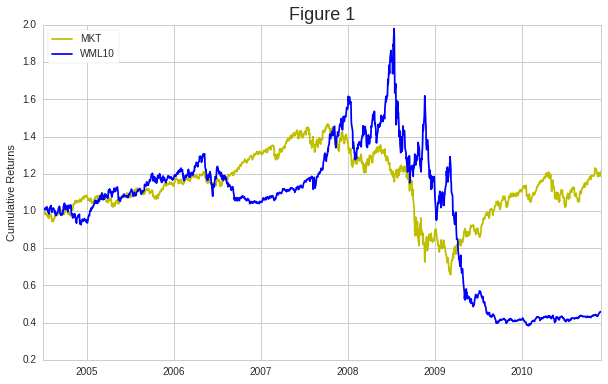## 3. Assessment of Momentum Underperformance with Winners and Losers Components¶

To gain insight into the behavior of WML10, we need to extend the analysis towards the consideration of the factor’s winning and losing components, W10 and L10, respectively. According to Figure 2, the spike in WML10 cumulative returns in mid-2008 was caused by L10 shifting downwards, resulting in a larger gap between winners and losers. Then, after the market began to regain strength and subsequently momentum crashed, the top and bottom 10% portfolios switched places: losers outperformed winners. Therefore, the momentum winners-minus-losers strategy became no longer profitable and resulted in going long the underperforming past winners while shorting overperforming past losers.

In :
w10 = mom_returns_1D
w10_cum = np.cumprod(w10 + 1)
l10 = mom_returns_1D
l10_cum = np.cumprod(l10 + 1)

plt.figure(figsize=(10,6))
plt.plot(days, mkt_cum/mkt_cum, color='y')
plt.plot(days, w10_cum/w10_cum, color='green')
plt.plot(days, l10_cum/l10_cum, color='blue')
plt.title('Figure 2', fontsize=18)
plt.ylabel('Cumulative Returns')
plt.legend(['MKT','W10','L10'],loc='upper left')
plt.show()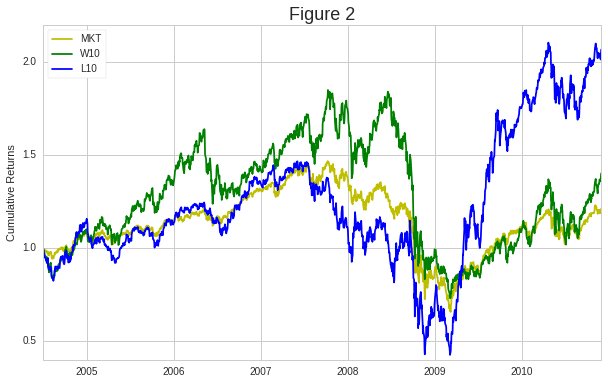## 4. Volatility-Timed Winners Approach¶

The momentum underperformance during the market downturn is addressed by different volatility-scaling approaches (e.g., Moskowitz, Ooi, Pedersen, 2012; Barroso and Santa-Clara, 2015). As will be shown, the market downturn broke the conceptual correlation between priors and forward returns. Therefore, the paper proposed to bypass this interval using volatility as a timing predictor and replacing scaling with the threshold function.

Defining the time window for calculating realized volatility

In :
vw = 126


Calculating realized volatility and volatility-timed winners

In :
w10_r2 = w10**2
w10_rv = np.sqrt(w10_r2.rolling(vw).sum()*2)

w10_scale = 0.27/w10_rv
w10_thrd = w10_scale.apply(lambda x: np.where(x < 1, 0, 1))
w10_timed = w10*w10_thrd


Comparing performance of the volatility-timed winners approach and the conventional winners-minus-losers momentum

In :
w10_timed_cum = np.cumprod(w10_timed[vw:]+1)

f, (ax1, ax2) = plt.subplots(2, sharex=True, sharey=False, gridspec_kw={'height_ratios': [3, 1]}, figsize=(10,6))

#Cumulative returns
ax1.plot(days[vw:], mkt_cum[vw:]/mkt_cum[vw], color='y', label="MKT")
ax1.plot(days[vw:], wml10_cum[vw:]/wml10_cum[vw], color="blue", label="WML10")
ax1.plot(days[vw:], w10_timed_cum/w10_timed_cum, color="green", label="W10-Timed")
ax1.set_ylabel('Cumulative Returns')
ax1.set_title('Figure 4', fontsize=18)
ax1.legend(loc='upper left')

#Annualized realized volatility
ax2.plot(days[vw:], w10_rv[vw:], color="red", label="W10 RV")
ax2.set_ylabel('Annual RV')
ax2.axhline(y=0.27, color='red', linestyle='--')
ax2.legend(loc='upper left')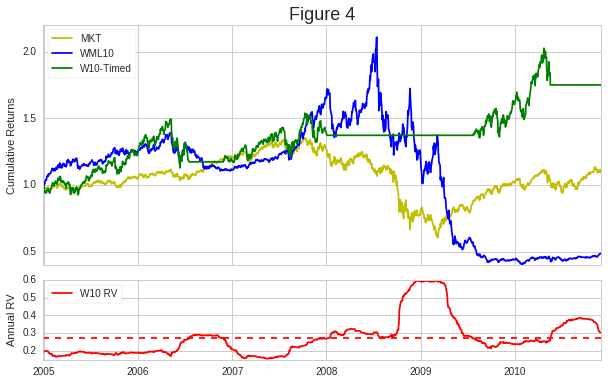## 5. Spearman Rank Correlation Analysis¶

The volatility-timed approach highlighted the relationship between the performance of the momentum factor and market downturn. Therefore, the momentum strategy was further investigated with Spearman rank correlation described in Lecture 23. The coefficients were computed between the 11 month momentum factor prior and 21-day forward returns. According to Table 1, this correlation during the 2005-2010 interval had a p-value of 0.6 and failed to reject the null hypothesis at alpha 1% of no monotonic relationship between the ranked variables. Then, as shown in Table 2, the volatility-timed winners approach resolved and enhanced the rank correlation between the momentum prior and forward returns by capturing and excluding the interval with the negative oscillations.

Table 1: Conventional Momentum Information Coefficient

In :
ic = al.performance.factor_information_coefficient(mom_data)
al.plotting.plot_information_table(ic[vw:])

Information Analysis

1D 21D
IC Mean 0.009 -0.003
IC Std. 0.149 0.173
t-stat(IC) 2.294 -0.568
p-value(IC) 0.022 0.570
IC Skew -0.202 -0.730
IC Kurtosis 0.287 0.747

Removing the volatility-timed intervals from the momentum data

In :
w10_rv_timed = w10_rv[vw:][w10_rv < 0.27]
ts= [pd.Timestamp(x).tz_localize('UTC') for x in w10_rv_timed.index.values]
mom_data_timed = mom_data.loc[ts]


Table 2: Volatility-Based Information Coefficient

In :
ic_timed = al.performance.factor_information_coefficient(mom_data_timed)
al.plotting.plot_information_table(ic_timed)

Information Analysis

1D 21D
IC Mean 0.015 0.030
IC Std. 0.126 0.133
t-stat(IC) 3.444 6.586
p-value(IC) 0.001 0.000
IC Skew -0.291 -0.615
IC Kurtosis 0.119 0.757

## 6. Comparison with Time Series Momentum and Risk-Based Asset Allocation¶

An alternative to the momentum factor is the time series momentum (TSMOM) strategy developed by Moskowitz, Ooi, and Pedersen (2012). The authors found the momentum effect in asset time series and applied it by computing the 12 month prior for each asset and then going long stocks with a positive overall prior return and short the negative counterparts. The asset-oriented ranking and selection was subsequently extended with volatility scaling. As discussed by Baltas (2015), TSMOM volatiltiy scaling represented a form of risk-based asset allocation (Lee, 2011). Risk parity (Qian, 2005; Maillard, Roncalli, Teiletche, 2010), one of these approaches, aims to equalize the risk contribution of each asset. When the pairwise correlations are ignored, the approach transforms into volatility parity (VP) allocation: \begin{equation} r*{t}^{VP}=\sum*{i=1}^{N*{t}}w*{t}^{i,VP}r*{t}^{i}, \mbox{ where } w*{t}^{i,VP}=\frac{1/\sigma*{t}^{i}}{\sum*{j=1}^{N*{t}}1/\sigma*{t}^{j}}, \forall i \end{equation} Using the VP returns, the long-only returns of the TSMOM strategy can be rewritten as follows: \begin{equation} r*{t}^{TSMOM,LO}=40\%\frac{\sum*{i=1}^{N*{t}}1/\sigma*{t}^{i}}{N*{t}}\sum*{i=1}^{N{t}}\frac{1/\sigma*{t}^{i}}{\sum*{i=1}^{N*{t}}1/\sigma*{t}^{i}}r*{t}^{i}=\frac{40\%}{\bar{\sigma}*{t}^{VP}}r*{t}^{VP} \end{equation} with weighted volatility: \begin{equation} \bar{\sigma}^{VP}=\sum*{i=1}^{N}w*{i}\sigma*{i}=\frac{N}{\sum*{i=1}^{N}1/\sigma*{i}} \end{equation}

Note: In place of the code used for the paper, the section shows a pipeline implemented from the IDE-based variant suggested by @Vladimir within this thread. In brief, the pipeline from Section 1 was extended with PercentileFilter which replaced Alphalens-based factor processing.

In :
#Compute inverse realized volatilities for input assets
class InvRV(CustomFactor):
inputs = [DailyReturns()]
window_length = 126

def compute(self, today, assets, out, returns):
ret_r2 = returns**2
ret_rv = np.sqrt(np.sum(ret_r2, axis=0)*2)
out[:] = 1./ret_rv

#Compute weighted volatility
class WV(CustomFactor):
inputs = [InvRV()]
window_length = 1

def compute(self, today, assets, out, inv_vols):
inv_vol_mean = np.nanmean(inv_vols)
wv = 1./inv_vol_mean
out[:] = np.ones(inv_vols.shape)*wv

#Compute weighted returns of VP10 portfolio
class VP10(CustomFactor):
inputs = [InvRV(), DailyReturns()]
window_length = 1

def compute(self, today, assets, out, inv_vols, returns):
inv_vol_mean = np.nanmean(inv_vols)
out[:] = (returns*inv_vols)/inv_vol_mean

#Compute weighted returns of TSMOM portfolio
class TSMOM10(CustomFactor):
inputs = [InvRV(), DailyReturns()]
window_length = 1

def compute(self, today, assets, out, inv_vols, returns):
tsmom = 0.4*returns*inv_vols
out[:] = tsmom

def make_pipeline():

pipe = Pipeline()

w10_filter = mom.percentile_between(90, 100)
pw10_filter = w10_filter & (mom > 0)

pipe.set_screen(universe)

return pipe

w10_pipeline = make_pipeline()

In :
results = run_pipeline(w10_pipeline, start_date, end_date)
results.dropna(inplace=True)


Pipeline Execution Time: 19.54 Seconds

Comparing weighted volatility with the momentum realized volatility from Section 4. In order to maintain consistency with the methodology from Section 4, weighted volatility were calculated using realized volatility for the top decile of winners. However, in contrast with the previous section, the VP10 weighted volatility was based only on the winners with positive prior returns to be consistent with the TSMOM long-only strategy. As shown in Figure 5 (and Exhibit 9), both portfolio volatilities captured the momentum crash interval, although VP10 weighted volatility at a higher level.

In :
w10_wv = results['wv'].mean(level=0)

plt.figure(figsize=(10,6))
plt.plot(days[vw:], w10_rv[vw:], color = 'lime')
plt.plot(days[vw:], w10_wv[vw:-21], color = 'blueviolet')
plt.title('Figure 5', fontsize=18)
plt.ylabel('Volatility')
plt.legend(['W10 Realized Volatility', 'VP10 Weighted Volatility'], loc = 'upper left')
plt.show()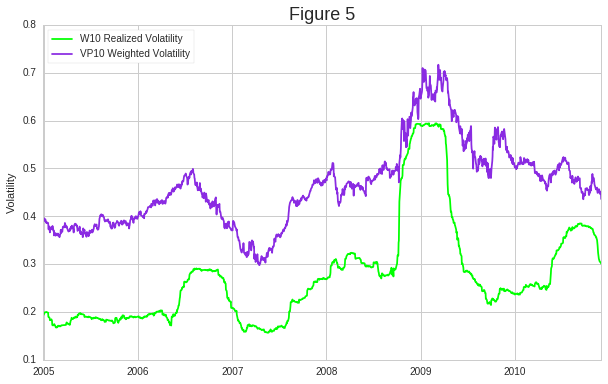Comparing performance of the winners portfolio (W10), volatility parity (VP10), time series momentum (TSMOM10), and volatility-timed winners approach (W10-Timed)

In :
vp10 = results['vp10'].mean(level=0)
tsmom10 = results['tsmom10'].mean(level=0)

vp10_cum = np.cumprod(vp10 + 1)
tsmom10_cum = np.cumprod(tsmom10 + 1)

plt.figure(figsize=(10,6))
plt.plot(days[vw:], w10_cum[vw:]/w10_cum[vw], color = 'y', label="W10")
plt.plot(days[vw:], vp10_cum[vw:-21]/vp10_cum[vw], color = 'blueviolet', label="VP10")
plt.plot(days[vw:], tsmom10_cum[vw:-21]/tsmom10_cum[vw], color = 'b', label="TSMOM10")
plt.plot(days[vw:], w10_timed_cum/w10_timed_cum, color = 'g', label="W10-Timed")
plt.title('Figure 6', fontsize=18)
plt.ylabel('Cumulative Returns')
plt.legend(loc = 'upper left')
plt.show()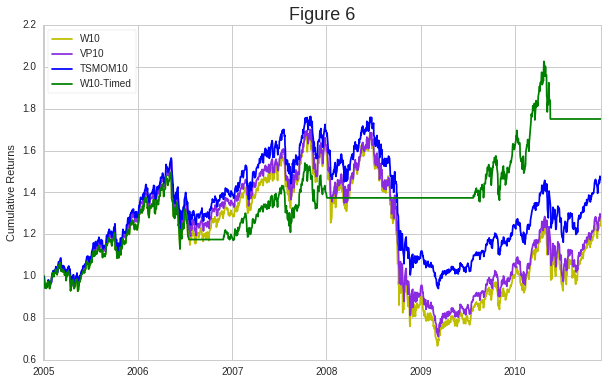## 7. Pyfolio Analysis¶

The section presents the summary statistics of the Pyfolio tearsheet (see Lecture 33: Portfolio Analysis) for the W10, VP10, TSMOM10, and W10-Timed approaches presented in Figure 6 and outlined in Section 3, Section 4, and Section 6.

#### 7.1 Top Decile Winners (W10) Summary Statistics¶

In :
pf.plotting.show_perf_stats(w10[vw:], mkt_returns[vw:])

Start date 2004-12-30 2010-12-01 71 4.2% 27.5% 29.8% 0.29 0.07 0.09 -60.5% 1.05 0.40 -0.30 5.17 0.95 -3.7% 0.05 0.80

#### 7.2 Volatility Parity (VP10) Summary Statistics¶

In :
pf.plotting.show_perf_stats(vp10[vw:], mkt_returns[vw:])

Start date 2004-12-30 2010-12-31 72 5.4% 37.0% 27.3% 0.33 0.09 0.07 -58.2% 1.06 0.45 -0.28 2.95 0.92 -3.4% 0.08 -0.11

#### 7.3 Time Series Momentum (TSMOM10) Summary Statistics¶

In :
pf.plotting.show_perf_stats(tsmom10[vw:], mkt_returns[vw:])

Start date 2004-12-30 2010-12-31 72 7.6% 55.4% 22.6% 0.44 0.16 0.00 -46.7% 1.08 0.61 -0.29 1.79 0.85 -2.8% 0.09 -0.09

#### 7.4 Volatility-Timed Winners Approach (W10-Timed) Summary Statistics¶

In :
pf.plotting.show_perf_stats(w10_timed[vw:], mkt_returns[vw:])

Start date 2004-12-30 2010-12-01 71 9.9% 74.6% 17.5% 0.63 0.40 0.77 -24.5% 1.15 0.88 -0.31 4.08 0.96 -2.2% 0.10 0.23

# Part II: Paper-Related Techniques and Extensions Proposed within this Forum¶

## 1. Deployment of VTW in IDE¶

IDE algorithm with the volatility-timed winners approach using DownsideVolatility as a timing predictor and bonds for high-volatility intervals.

In [ ]:
'''
# Volatility-Timed Momentum Winners Plus Bonds
from quantopian.pipeline.factors import CustomFactor, DailyReturns
from quantopian.algorithm import attach_pipeline, pipeline_output
from quantopian.pipeline.data.builtin import USEquityPricing
from quantopian.pipeline import Pipeline
from scipy.stats.mstats import winsorize
import quantopian.optimize as opt
import numpy as np
import math
# ----------------------------------------------------------------------
STK_SET = QTradableStocksUS(); MOM = 252; EXCL = 21; W = 0.01; PCTL = 10
VOL = 126; TRHD = 0.27; LEV = 1.0; BONDS = symbols('TLT', 'IEF')
# ----------------------------------------------------------------------
def initialize(context):
schedule_function(rebalance, date_rules.every_day(), time_rules.market_open(minutes = 1))
mom_w = mom.winsorize(W, 1.- W)
longs = mom_w.percentile_between(100 - PCTL, 100)
columns = {'longs': longs, 'vol': vol}
screen = longs & mom.notnull() & mom.notnan() & mom.isfinite()
attach_pipeline(Pipeline(columns, screen ), 'Yulia_pipe')

def rebalance(context, data):
wt = {}; wt_bnd = LEV
output = pipeline_output('Yulia_pipe')
vol = output.vol.mean()
ratio = vol / TRHD
record(ratio = ratio, thrd = 1.0 )
longs = output[output.longs].index
wt_long = LEV/len(longs) if ratio < 1.0 else 0.0
for sec in context.portfolio.positions:
if sec not in longs and sec not in BONDS: wt[sec] = 0
for sec in longs: wt[sec] = wt_long; wt_bnd -= wt[sec]
for sec in BONDS: wt[sec] = wt_bnd / len(BONDS)
order_optimal_portfolio(opt.TargetWeights(wt), [opt.MaxGrossExposure(LEV)])

record(leverage = context.account.leverage)
print len(context.portfolio.positions)

class Momentum(CustomFactor):
""" Conventional Momentum factor """
inputs = [USEquityPricing.close]
window_length = MOM
def compute(self, today, assets, out, prices):
out[:] = (prices[-EXCL] - prices[-MOM])/prices[-MOM]

class Downside_Volatility(CustomFactor):
inputs = [DailyReturns()]
window_length = VOL
def compute(self, today, assets, out, returns):
returns[returns > 0] = np.nan
down_vol = np.nanstd(returns, axis = 0)
ann_down_vol = down_vol*math.sqrt(252)
out[:] = ann_down_vol
'''


Load backtest data generated by the above algorithm

In :
bt = get_backtest('5d47310d7634f462119643e4')
returns = bt.daily_performance['returns']

100% Time: 0:03:12|###########################################################|


Plot Cumulative Returns

In :
cum_returns = ep.cum_returns(returns)
ax = cum_returns.plot(figsize=(14,5))
ax.set(title='Cumulative Returns', ylabel='returns', xlabel='date');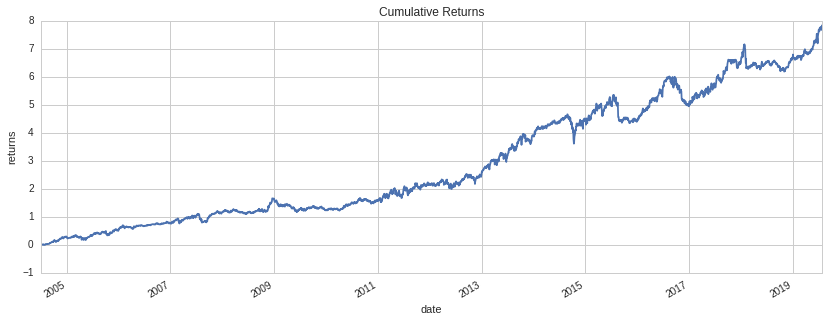Print summary statistics of Pyfolio tearsheet

In :
benchmark_rets = pf.utils.get_symbol_rets('SPY')
pf.plotting.show_perf_stats(returns, benchmark_rets)

Start date 2004-07-01 2019-07-26 180 15.6% 783.8% 15.0% 1.04 0.84 0.98 -18.6% 1.20 1.47 -0.40 2.04 0.97 -1.8% 0.15 0.09

## 2. Application of VIX Data for Selecting and Highlighting the High/Low Volatility Regimes¶

In :
start_date = returns.index
end_date = returns.index[-1]

In :
# Load VIX data (https://www.quantopian.com/data/quandl/cboe_vix)
from quantopian.interactive.data.quandl import cboe_vix
from odo import odo

vix_data = (odo(cboe_vix[['vix_close', 'asof_date']], pd.DataFrame)
.set_index('asof_date')
.rename_axis('date')
.tz_localize('utc')
.sort_index()
.loc[start_date:end_date]
.vix_close)

In :
# Plot VIX timeseries
ax = vix_data.plot(color='black', figsize=(15, 5))

# The threshold for dividing high/low vol regimes is the long-term mean of S&P 500 volatility.
threshold = 20 # 18, 20

# Highlight regions of high volatility.
x = vix_data.index
ymax = vix_data.max() + 5
ax.fill_between(x, 0, ymax, where=vix_data > threshold, facecolor='red', alpha=0.5, interpolate=True)

ax.set_xlim([x, x[-1]])
ax.set_ylim([0, ymax])
ax.set(title='Volatility Index', ylabel='vix');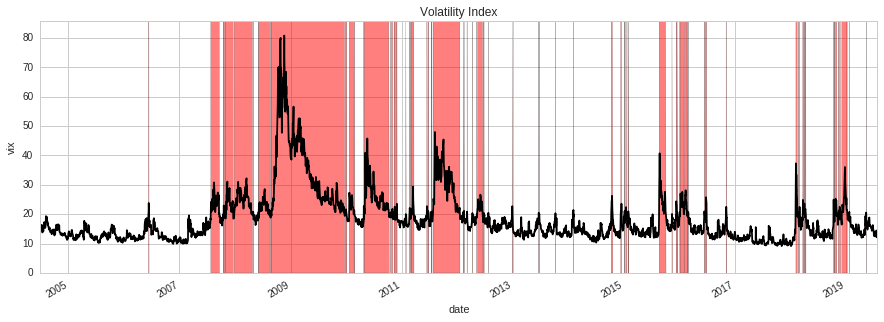In :
# Boolean series containing True for high vol regimes, and False for low-vol regimes.
regimes = (vix_data >= threshold)


## 3. Plotting Cumulative Returns in Different Regimes¶

In :
def plot_cumulative_returns_by_regime(returns, regimes):
"""Plot cumulative returns generated within each regime.
Parameters
----------
returns : pd.Series[float]
Timeseries of algorithm returns.
regimes : pd.Series[bool]
Boolean series indicating whether each day was high or low volatility.
"""
fig, (original_ax, split_ax) = plt.subplots(ncols=2, nrows=1, figsize=(15, 5))
cum_rets = ep.cum_returns(pd.DataFrame({
'Returns': returns,
'High Volatility': returns.where(regimes, 0),
'Low Volatility': returns.where(~regimes, 0),
}))

# Plot algo's cumulative returns on the left axis.
pd.concat([cum_rets['Returns'].where(regimes, np.nan).rename('High Volatility'),
cum_rets['Returns'].where(~regimes, np.nan).rename('Low Volatility')],
axis=1).plot(ax=original_ax, title="Algorithm's Cumulative Returns")

# Plot cumulative returns within each regime on the right axis.
title = "Algorithm's Returns when Trading in High/Low Volatility Regime"
cum_rets[['High Volatility', 'Low Volatility']].plot(ax=split_ax, title=title)

plot_cumulative_returns_by_regime(returns, regimes)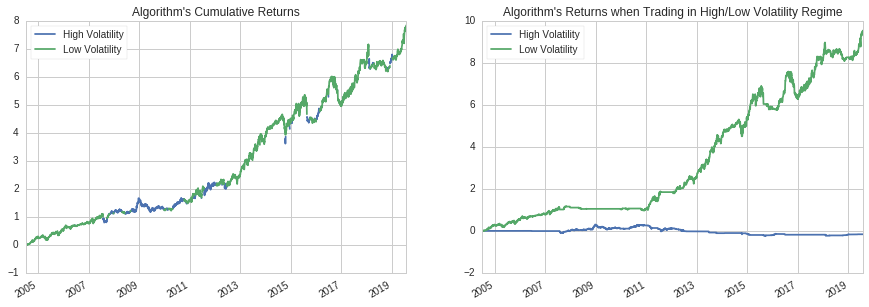## 4. Sharpe Ratio Bootstrap Test¶

In :
# function used for bootstrap: get random test dates.
def random_date_ranges(date_index, segments_lengths):
"""
Generate bootstrap samples with same segment numbers
and lengths over the entire data range.

Parameters
----------
date_index : pd.Series
The data range used for bootstrap test.
segments_lengths : list
The numbers of consecutive days in a selected high vol/low vol regime.

Returns
-------
test_dates_index : pd.Series
Index series for bootstrap.
"""

# Generate bootstrap random samples from the entire data range.
total_segments_length = sum(segments_lengths)
total_gaps_length = len(date_index) - total_segments_length
gaps_lengths = random_partition(total_gaps_length, len(segments_lengths) + 1)
# shuffle the segments' lengths of the selected regime
np.random.shuffle(segments_lengths)

samples = []
cursor = 0
for gap, segment in zip(gaps_lengths, segments_lengths):
cursor += gap
samples.append(date_index[cursor:cursor + segment])
cursor += segment
return pd.Index(np.hstack(samples), tz='UTC')

# function used for getting random test dates.
def random_partition(N, k):
"""
Randomly generate a partition of the integer N into k pieces.
"""
samples = np.sort(np.random.choice(N + 1, replace=False, size=k - 1))
lengths = np.hstack([samples, np.diff(samples), N - samples[-1]])
assert lengths.sum() == N, lengths.sum()

return lengths.tolist()

# function used for bootstrap: count consecutive days in high/low vol range.
def reference_regime_segments(selected_regime_data):
'''
Count consecutive days in each high/low vol range and store the number.

Parameters
----------
selected_regime_data : pd.Series
Referece data from a selected regime.

Returns
-------
segments_lengths : pd.Series
A series of numbers of consecutive days in a selected regime.
'''
lengths = (selected_regime_data.groupby((~selected_regime_data).cumsum())
.sum()
.astype(int))
# Remove possible leading and trailing zeros.
segment_lengths = list(lengths.loc[lengths != 0].values)
return segment_lengths

def compute_percentile_score(returns, selection, output=True, seed=0):
'''
Compute percentile score for selected Sharpe ratio in random Sharpe ratio distribution.

Parameters
----------
returns : pd.Series[float]
Timeseries of algorithm returns.
selection : pd.Series[bool]
Boolean series indicating whether each day was in the selected regime or not.
output :  bool (optional)
Whether to output the variables of sharpe_ratio_selected_regime and random_sharpe_ratios.
seed : int, optional
Seed to use for random number generation.

Returns
-------
score : float
The percentile score for selected Sharpe ratio.
sharpe_ratio_selected_regime : float
The Sharpe ratio of returns in the selected regime
random_sharpe_ratios:  pd.Series
Samples' sharpe ratios
'''
rng = np.random.RandomState(seed)
random_sharpe_ratios = []
num_samples = 1000

# Sharpe ratio of algorithm returns on selected days.
sharpe_ratio_selected_regime = ep.sharpe_ratio(returns.loc[selection])

# Compute the segments' lengths in the selected regime
segments_lengths = reference_regime_segments(selection)

for j in range(num_samples):
test_dates = random_date_ranges(returns.index, segments_lengths)
random_sharpe_ratios.append(ep.sharpe_ratio(returns.loc[test_dates]))

# compute the percentile of sharpe_ratio_selected_vol
# in Sharpe ratio distribution generated by bootstrapping.
score = st.percentileofscore(random_sharpe_ratios,
sharpe_ratio_selected_regime,
kind='strict')
if output:
random_sharpe_ratios = pd.Series(random_sharpe_ratios)
return score, sharpe_ratio_selected_regime, random_sharpe_ratios
else:
return score
return score

In :
def regime_sharpe_ratio(returns, regimes):
'''
Compute Sharpe ratios for different regimes.

Parameters
----------
returns : pd.Series[float]
Series containing daily algorithm returns.
regimes: pd.Series[bool]
Series containing True/False values indicating whether a given day was
high or low volatility.
'''
regime_sharpe_ratio = {}
if regimes.all():
raise ValueError(
"The reference data does not involve any low volatility dates."
"Please try to run the analysis with longer backtest period."
)

if (~regimes).all():
raise ValueError(
"The reference data does not involve any high volatility' dates."
"Please try to run the analysis with longer backtest period."
)

regime_sharpe_ratio['high_vol'] = ep.sharpe_ratio(returns.loc[regimes])
regime_sharpe_ratio['low_vol'] = ep.sharpe_ratio(returns.loc[~regimes])
return pd.Series(regime_sharpe_ratio)

In :
sharpe_ratios = regime_sharpe_ratio(returns, regimes)

# Compute Sharpe ratio over entire data range.
overall_sharpe_ratio = ep.sharpe_ratio(returns)

In :
import scipy.stats as st

if sharpe_ratios['high_vol'] >= sharpe_ratios['low_vol']:
print("Computing percentile scores for high vol regime...")
selection = regimes
else:
print("Computing percentile scores for low vol regime...")
selection = ~regimes
(score,
sharpe_ratio_selected_regime,
random_sharpe_ratios) = compute_percentile_score(returns, selection, output=True)
print "Done!"

Computing percentile scores for low vol regime...
Done!

In :
# Plot Sharpe ratio distribution and mark the sharpe_ratio_selected_regime position in the distribution.
ax = pd.Series(random_sharpe_ratios).plot.hist(bins=30, color='paleturquoise', figsize=(8,5))
ax.axvline(sharpe_ratio_selected_regime, color='b', linestyle='dashed', linewidth=2)

ax.text(sharpe_ratio_selected_regime,
ax.get_ylim()+50,
"sharpe_ratio_selected_regime: \n{}".format(sharpe_ratios.argmax()+' preferred'),
horizontalalignment='center',
verticalalignment='center',
bbox=dict(facecolor='white', alpha=0.9))
ax.set(title="Sharpe Ratios' Distribution", xlabel='sharpe ratio');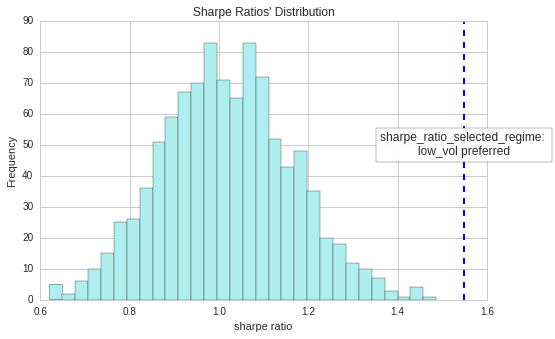In :
pd.Series({'algo_pattern' : sharpe_ratios.argmax()+' preferred',
'overall_sharpe_ratio' : overall_sharpe_ratio,
'sharpe_ratio_high_vol' : sharpe_ratios['high_vol'],
'sharpe_ratio_low_vol' : sharpe_ratios['low_vol'],
'sharpe_ratio_preferred_vol' : sharpe_ratios.max(),
'percentile_score': score}).to_frame('vol_regime_analysis')

Out:
vol_regime_analysis
algo_pattern low_vol preferred
overall_sharpe_ratio 1.03877
percentile_score 100
sharpe_ratio_high_vol -0.186573
sharpe_ratio_low_vol 1.54923
sharpe_ratio_preferred_vol 1.54923